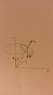# Gyroscope- Angular Momentum and Torque

• Fizzios

#### Fizzios

I was reading on gyroscopes, and everything seemed to make sense: the spin angular momentum along the axis of the gyroscope changes due to the torque by gravity, causing precession. However, I can't understand why we are measuring angular momentum (the spin of the gyroscope) from the center of the spinning disk, but the torque from the origin (pivot point). Aren't we supposed to measure torque and angular momentum about the same point to use the relation Torque = dL/dt?

Some clarification would be much appreciated!

#### Attachments

•gyroscope.jpg
5.1 KB · Views: 588

For an object with no net linear momentum (e.g. a gyroscope spinning more or less in place), its angular momentum will not depend on what reference point you choose as the origin.

For a torque being applied by a "couple" (a pair of equal but opposite forces that act on parallel lines rather than on the same line, e.g. N and W), it does not matter what reference point you use to compute the net torque.

Or you can avoid the problem and compute the torque produced from the upward force N using a reference point at the center of mass of the gyroscope.

Thanks, I see. So it would be safe to say that you get the same change in angular momentum measured anywhere along the axis of the gyroscope?

Also, hypothetically, in a gravity-free environment, if someone were to push a spinning gyroscope at the pivot (acting like the normal force), would it produce the same precession motion? Or is a torque couple needed?

Yes, the angular momentum for an object spinning around an axis is the same no matter where on the axis you choose to measure it from.

In a gravity-free environment the gyroscope will be accelerating in the direction of the net force. But you can choose to analyze the system from an accelerating frame in which the center of mass is motionless (like in an elevator that is accelerating upward). In this frame there is a downward inertial force that acts just like gravity. So you can have a force couple if you want one.